/

### Blending Independent Components and Principal Components Analysis 3.1 Characteristics of PCA

Next page

3.1          Characteristics of PCA

Earlier, we noted some similarities between ICA and PCA but also noted some differences. In particular, ICA focuses on ‘independence’, ‘non-Normality’ and ‘lack of complexity’ and assumes that source signals exhibit these features, whereas PCA focuses merely on lack of correlatedness. Indeed, PCA analysis will even ‘unmix’ pure Gaussian (i.e. normally distributed) signals. Or rather, it will decompose multiple Gaussian output signals into some presumed orthogonal Gaussian input signals, which can be ordered with ones higher up the ordering explaining more of the variability in the output signal ensemble than ones lower down the ordering.

PCA involves calculating the eigenvectors and eigenvalues of the covariance matrix, i.e. the matrix of correlation coefficients between the different signals. Usually, it would be assumed that the covariance matrix had been calculated in a manner that gives equal weight to each data point. However, this is not essential; we could equally use a computation approach in which different weights were given to different data points, e.g. an exponentially decaying weighting that gives greater weight to more recent observations.

Fordifferent output signals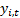, with covariance matrix,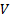, between the output signals, PCA searches for the(some possibly degenerate) eigenvectors,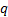, satisfying the following matrix equation for some scalar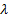.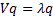For a non-negative definite symmetric matrix (asshould be if it actually corresponds to a covariance matrix), thevalues ofare all non-negative. We can therefore order the eigenvalues in descending order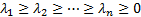. The corresponding eigenvectors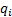are orthogonal i.e. have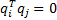if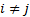and are also typically normalised so that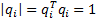(i.e. so that they have ‘unit length’). For any’s that are equal, we need to choose a corresponding number of orthonormal eigenvectors that span the relevant subspace.

Bywe mean the vector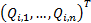such that the signal corresponding to eigenvalue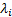is expressible as: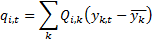If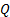is the matrix with coefficients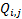then the orthonomalisation convention adopted above means that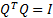whereis the identity matrix. This means that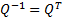and hence we may also write the output signals as a linear combination of the eigenvector signals as follows (in each case up to a constant value, since the covariances do not depend on means of series):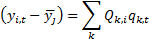Additionally, we have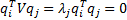ifand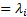if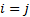. We also note that if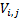are the coefficients ofthen each individualis (here assuming that we have been using ‘sample’ rather than ‘population’ values for variances: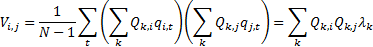Hence the sum of the variances of each output signal, i.e. the trace of the covariance matrix, satisfies: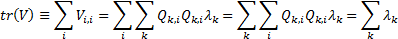We can interpret this as indicating that the aggregate variability of the output signals (i.e. the sum of their individual variabilities) is equal to the sum of the eigenvalues. Hence the larger the eigenvalue the more the corresponding eigenvector signal ‘contributes’ to the aggregate variability across the ensemble of possible output signals.